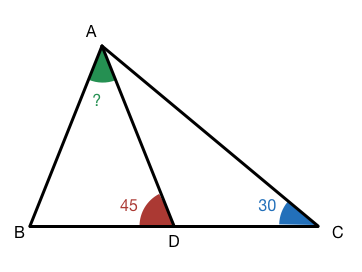# You'll be stuck

Geometry Level 3In $$\triangle ABC$$, let $$D$$ be the midpoint of $$\overline{BC}$$. If $$\angle ADB = 45^\circ$$ and $$\angle ACD = 30^\circ$$, what is $$\angle BAD$$ in degrees?

×

Problem Loading...

Note Loading...

Set Loading...